Updating search results...

# 771 Results

View
Selected filters:
• remix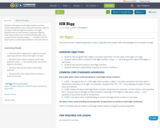Conditional Remix & Share Permitted
CC BY-NC-SA
Rating
0.0 stars

Students will explore multi-digit numbers and the relationship between ones, tens and hundreds; a digit in one place is 10x the digit in the place to its right. Students will use their bodies to represent digits in multi-digit numbers up to the hundredths place and compare these numbers using <, =, >. Students will use their bodies as multi-digit numbers to add and subtract.

Subject:
Mathematics
Material Type:
Activity/Lab
Teaching/Learning Strategy
08/08/2019Unrestricted Use
CC BY
Rating
0.0 stars

Students will explore multi-digit numbers and the relationship between ones, tens and hundreds; a digit in one place is 10x the digit in the place to its right. Students will use their bodies to represent digits in multi-digit numbers up to the hundredths place and compare these numbers using <, =, >. Students will use their bodies as multi-digit numbers to add and subtract.
Thanks
http://driverrestore.com/

Subject:
Mathematics
Material Type:
Activity/Lab
Lesson Plan
Teaching/Learning Strategy
08/08/2019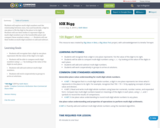Conditional Remix & Share Permitted
CC BY-NC-SA
Rating
0.0 stars

Students will explore multi-digit numbers and the relationship between ones, tens and hundreds; a digit in one place is 10x the digit in the place to its right. Students will use their bodies to represent digits in multi-digit numbers up to the hundredths place and compare these numbers using <, =, >. Students will use their bodies as multi-digit numbers to add and subtract.

Subject:
Mathematics
Material Type:
Activity/Lab
Teaching/Learning Strategy
08/08/2019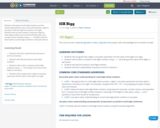Conditional Remix & Share Permitted
CC BY-NC-SA
Rating
0.0 stars

Students will explore multi-digit numbers and the relationship between ones, tens and hundreds; a digit in one place is 10x the digit in the place to its right. Students will use their bodies to represent digits in multi-digit numbers up to the hundredths place and compare these numbers using <, =, >. Students will use their bodies as multi-digit numbers to add and subtract.

Subject:
Mathematics
Material Type:
Activity/Lab
Teaching/Learning Strategy
08/08/2019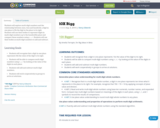Conditional Remix & Share Permitted
CC BY-NC-SA
Rating
0.0 stars

Students will explore multi-digit numbers and the relationship between ones, tens and hundreds; a digit in one place is 10x the digit in the place to its right. Students will use their bodies to represent digits in multi-digit numbers up to the hundredths place and compare these numbers using <, =, >. Students will use their bodies as multi-digit numbers to add and subtract.

Subject:
Mathematics
Material Type:
Activity/Lab
Teaching/Learning Strategy
08/08/2019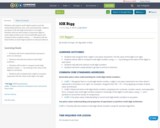Conditional Remix & Share Permitted
CC BY-NC-SA
Rating
0.0 stars

Students will explore multi-digit numbers and the relationship between ones, tens and hundreds; a digit in one place is 10x the digit in the place to its right. Students will use their bodies to represent digits in multi-digit numbers up to the hundredths place and compare these numbers using <, =, >. Students will use their bodies as multi-digit numbers to add and subtract.

Subject:
Mathematics
Material Type:
Activity/Lab
Teaching/Learning Strategy
08/08/2019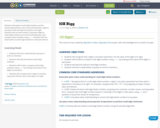Conditional Remix & Share Permitted
CC BY-NC-SA
Rating
0.0 stars

Students will explore multi-digit numbers and the relationship between ones, tens and hundreds; a digit in one place is 10x the digit in the place to its right. Students will use their bodies to represent digits in multi-digit numbers up to the hundredths place and compare these numbers using <, =, >. Students will use their bodies as multi-digit numbers to add and subtract.

Subject:
Mathematics
Material Type:
Activity/Lab
Teaching/Learning Strategy
08/08/2019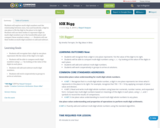Conditional Remix & Share Permitted
CC BY-SA
Rating
0.0 stars

Students will explore multi-digit numbers and the relationship between ones, tens and hundreds; a digit in one place is 10x the digit in the place to its right. Students will use their bodies to represent digits in multi-digit numbers up to the hundredths place and compare these numbers using <, =, >. Students will use their bodies as multi-digit numbers to add and subtract.

Subject:
Mathematics
Material Type:
Activity/Lab
Teaching/Learning Strategy
08/08/2019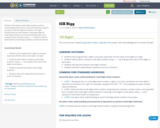Only Sharing Permitted
CC BY-NC-ND
Rating
0.0 stars

Students will explore multi-digit numbers and the relationship between ones, tens and hundreds; a digit in one place is 10x the digit in the place to its right. Students will use their bodies to represent digits in multi-digit numbers up to the hundredths place and compare these numbers using <, =, >. Students will use their bodies as multi-digit numbers to add and subtract.

Subject:
Mathematics
Material Type:
Activity/Lab
Teaching/Learning Strategy
08/08/2019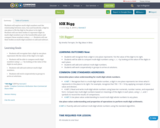Conditional Remix & Share Permitted
CC BY-NC-SA
Rating
0.0 stars

Students will explore multi-digit numbers and the relationship between ones, tens and hundreds; a digit in one place is 10x the digit in the place to its right. Students will use their bodies to represent digits in multi-digit numbers up to the hundredths place and compare these numbers using <, =, >. Students will use their bodies as multi-digit numbers to add and subtract.

Subject:
Mathematics
Material Type:
Activity/Lab
Teaching/Learning Strategy
08/08/2019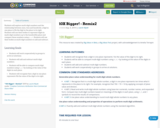Conditional Remix & Share Permitted
CC BY-NC-SA
Rating
0.0 stars

Students will explore multi-digit numbers and the relationship between ones, tens and hundreds; a digit in one place is 10x the digit in the place to its right. Students will use their bodies to represent digits in multi-digit numbers up to the hundredths place and compare these numbers using <, =, >. Students will use their bodies as multi-digit numbers to add and subtract.

Subject:
Mathematics
Material Type:
Activity/Lab
Teaching/Learning Strategy
08/08/2019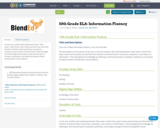Conditional Remix & Share Permitted
CC BY-NC-SA
Rating
0.0 stars

In this unit, students will understand where “fake news” comes from, why it exists and how they can think like fact checkers to become fluent consumers, evaluators, and creators of information. They will apply this knowledge by selecting a controversial topic to evaluate, synthesize, and analyze all aspects before sharing with a local audience.

Subject:
Education
Material Type:
Activity/Lab
Assessment
Homework/Assignment
Lesson
Lesson Plan
Teaching/Learning Strategy
Unit of Study
08/08/2019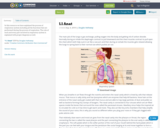Conditional Remix & Share Permitted
CC BY-NC
Rating
0.0 stars

In this resource we have explained the process of respiratory system including all the functions that are performed during the process of respiration. The role of each and every part involved in respiratory system is explained with proper diagrams as well.

Subject:
Life Science
Material Type:
Diagram/Illustration
Lecture Notes
Lesson
08/08/2019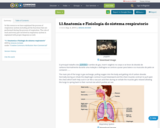Conditional Remix & Share Permitted
CC BY-NC
Rating
0.0 stars

In this resource we have explained the process of respiratory system including all the functions that are performed during the process of respiration. The role of each and every part involved in respiratory system is explained with proper diagrams as well.

Subject:
Life Science
Material Type:
Diagram/Illustration
Lecture Notes
Lesson
08/08/2019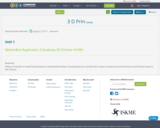Conditional Remix & Share Permitted
CC BY-NC
Rating
0.0 stars

Using a 3 D printer to model the printing of a mathematical object. Continueing on to calculate the volume of material used and total cost if the item based on this volume.

Subject:
Applied Science
Material Type:
Lesson
08/08/2019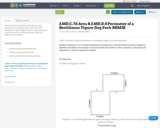Conditional Remix & Share Permitted
CC BY-NC-SA
Rating
0.0 stars

This task requires students to find the area and perimeter of a rectilinear figure and model their thinking through multiple equations. This task relates to the Common Core State Standards: 3.MD.C.7d, 3.MD.D.8, and 3.OA.D.8.

Subject:
Education
Mathematics
Material Type:
Activity/Lab
Assessment
Homework/Assignment
05/06/2020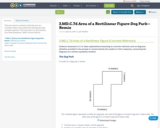Conditional Remix & Share Permitted
CC BY-NC-SA
Rating
0.0 stars

This task requires students to find the area of a rectilinear figure and model their thinking through multiple equations. This task relates to the Common Core State Standards: 3.MD.C.7d and 3.OA.D.8.

Subject:
Education
Mathematics
Material Type:
Activity/Lab
Assessment
Homework/Assignment